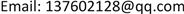1. 引言

2. 峰值电流的采样电路

3. 基于峰值电流之和的MPPT扰动观察法

P p v = U i ⋅ I i = 2 π ⋅ U i ⋅ I p _ i ( k ) (1)

I p _ i ( k ) = 1 2 ⋅ t o n ⋅ I p k _ i ( k ) T s (2)

t o n = L p I p k _ i ( k ) U i (3)

P i = I i ⋅ U i = L m ⋅ I p k _ i 2 ( k ) π ⋅ T s (4)

I t o t a l = ∑ i = 1 N I p k _ i ( k ) = k c o f f ⋅ I p k _ max ( k ) (5)

4. 基于占空比观测器的控制方法4.1. 反激变换器开关管占空比观测器设计原理

i s _ p e a k = N ⋅ i p _ p e a k (6)

i p _ p e a k = V i n L p ⋅ t o n (7)

i s _ p e a k = V o u t ( 1 / N ) 2 L p ⋅ t o f f (8)

N为变压器原边与副边匝数比。实际图3中的负载为倒相逆变器，其两端电压为Vout，输出电流即为并网电流的绝对值，设电网电压 v g r i d = V g m ⋅ sin ( ω t ) ，电网电流 i g = I g m ⋅ sin ( ω t ) 。

1) 临界连续模式

t o n _ B C M = L p ⋅ i p k _ i ( k ) V p v (9)

t o f f _ B C M = L p ⋅ i p k _ i ( k ) V o u t ⋅ N 2 (10)

i s _ a v g _ B C M ( ω t ) = N ⋅ i _ r e f _ B C M ( ω t ) ⋅ t o f f _ B C M ( ω t ) 2 ⋅ [ t o n _ B C M ( ω t ) + t o f f _ B C M ( ω t ) ] (11)

i s _ a v g _ B C M ( ω t ) = I g m _ r e f sin ( ω t ) (12)

i p k _ i ( k ) = 2 ⋅ I g m _ r e f ⋅ [ V o u t ⋅ | sin ( ω t ) | V p v + | sin ( ω t ) | N ] (13)

2) 断续导通模式

t o f f _ D C M = L p ⋅ i p k _ i ( k ) V o u t ⋅ N 2 (14)

i s _ a v g _ D C M ( ω t ) = 0.5 ⋅ N ⋅ i r e f _ D C M ( ω t ) ⋅ t o f f _ D C M ( ω t ) T D C M (15)

i s _ a v g _ D C M ( ω t ) = I g m _ r e f ⋅ sin ( ω t ) (16)

i p k _ i ( k ) = 2 ⋅ I g m _ r e f ⋅ T D C M ⋅ V o u t ⋅ N L p ⋅ | sin ( ω t ) | (18)

4.2. 并网微逆变器的开环控制策略

5. 实验分析

Main performance indicators of dual-channel interleaved flyback photovoltaic grid-connected micro-inverter

6. 结论